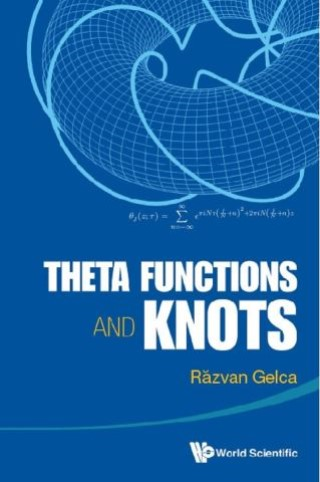## Theta Functions And Knots• Description
• Author
• Info
• Reviews

### Description

This book presents the relationship between classical theta functions and knots. It is based on a novel idea of Răzvan Gelca and Alejandro Uribe, which converts Weil's representation of the Heisenberg group on theta functions to a knot theoretical framework, by giving a topological interpretation to a certain induced representation. It also explains how the discrete Fourier transform can be related to 3- and 4-dimensional topology.

Theta Functions and Knots can be read in two perspectives. Readers with an interest in theta functions or knot theory can learn how the two are related. Those interested in Chern–Simons theory will find here an introduction using the simplest case, that of abelian Chern–Simons theory. Moreover, the construction of abelian Chern–Simons theory is based entirely on quantum mechanics and not on quantum field theory as it is usually done.

Both the theory of theta functions and low dimensional topology are presented in detail, in order to underline how deep the connection between these two fundamental mathematical subjects is. Hence the book is self-contained with a unified presentation. It is suitable for an advanced graduate course, as well as for self-study.

Contents:
• Prologue
• A Quantum Mechanical Prototype
• Surfaces and Curves
• The Theta Functions Associated to a Riemann Surface
• From Theta Functions to Knots
• Some Results About 3- and 4-Dimensional Manifolds
• The Discrete Fourier Transform and Topological Quantum Field Theory
• Theta Functions in the Quantum Group Perspective
• An Epilogue — Abelian Chern–Simons Theory

Readership: Graduate students and young researchers with an interest in complex analysis, mathematical physics, algebra geometry and low dimensional topology.